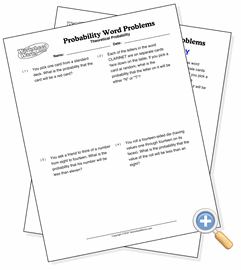# Theoretical ProbabilitySolve probability word problems involving combinations

Theoretical probability is the ratio between the number of ways an event can occur and the total number of possible outcomes in the sample space. Put simply, it’s the chance that something will happen, usually expressed as a percentage.